## Example Questions

← Previous 1

### Example Question #1 : How To Find The Area Of A Rectangle

The length of a rectangle is 5 times its width. Its width is 3 inches long. What is the area of the rectangle in square inches?

36
75
45
15
Explanation:

The length is 5 x 3 = 15 inches. Multiplied by the width of 3 inches, yields 45 in2.

### Example Question #2 : How To Find The Area Of A Rectangle

A rectangle’s base is twice its height.  If the base is 8” long, what is the area of the rectangle?

12 in2

32 in2

16 in2

64 in2

24 in2

32 in2

Explanation:

Rectangle

B = 2H

B = 8”

H = B/2 = 8/2 = 4”

Area = B x H = 8” X 4” = 32 in2

### Example Question #3 : How To Find The Area Of A Rectangle

The length of a rectangle is two more than twice the width. The perimeter is 58ft. What is the area of the rectangle?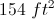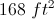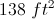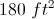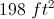Explanation:

For a rectangle,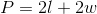and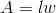, whereis the length and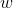is the width.

Letbe equal to the width. We know that the length is equal to "two more than twice with width."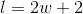The equation to solve for the perimeter becomes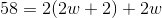.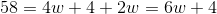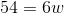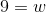Now that we know the width, we can solve for the length.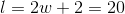Now we can find the area using.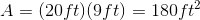### Example Question #1 : How To Find The Area Of A Rectangle

A rectangle has a perimeter of 40 inches.  It is 3 times as long as it is wide.  What is the area of the rectangle in square inches?

75

45

60

86

75

Explanation:

The width of the rectangle is w, therefore the length is 3w.  The perimeter, P, can then be described as P = w + w + 3w +3w

40 = 8w

w = 5

width = 5, length = 3w = 15

A = 5*15 = 75 square inches

### Example Question #5 : How To Find The Area Of A Rectangle

Angela is carpeting a rectangular conference room that measures 20 feet by 30 feet. If carpet comes in rectangular pieces that measures 5 feet by 4 feet, how many carpet pieces will she need to carpet the entire room?

30

31

600

20

29

30

Explanation:

First, we need to find the area of the room. Because the room is rectangular, we can multiply 20 feet by 30 feet, which is 600 square feet. Next, we need to know how much space one carpet piece covers. Because the carpet pieces are also rectangular, we can multiply 4 feet by 5 feet to get 20 feet. To determine how many pieces of carpet Angela will need, we must divide the total square footage of the room (600 feet) by the square footage covered by one carpet piece (20 feet). 600 divided by 20 is 30, so Angela will need 30 carpet pieces to carpet the entire room.

### Example Question #6 : How To Find The Area Of A Rectangle

If the width of a rectangle is 8 inches, and the length is half the width, what is the area of the rectangle in square inches?

12

32

16

64

20

32

Explanation:

the length of the rectangle is half the width, and the width is 8, so the length must be half of 8, which is 4.

The area of the rectangle can be determined from multiplying length by width, so,

4 x 8 = 32 inches squared

### Example Question #7 : How To Find The Area Of A Rectangle

If Mrs. Stietz has a patio that measures 96 inches by 72 inches and she wants to cover it with stone tiles that measure one foot by half a foot, what is the minimum number of tiles she needs to cover the patio?

6912

48

96

12

14

96

Explanation:

96. Converting the dimensions of the tiles to inches, they each measure 12 inches by 6 inches.  This means that there need to be 8 tiles to span the length of the patio, and 6 tiles to span the width of the patio.  She needs to cover the entire area, so we can multiply 8 times 12 to get 96, the number of tiles she needs for the patio.

### Example Question #1 : How To Find The Area Of A Rectangle

Mark is making a plan to build a rectangular garden.  He has 160 feet of fence to form the outside border of the garden.  He wants the dimensions to look like the plan outlined below: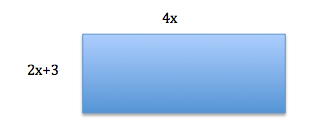What is the area of the garden, rounded to the nearest square foot?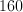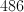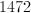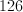Explanation:

Perimeter:  Sum of the sides:

4x + 4x + 2x+8 +2x+8 = 160

12x + 6 = 160

12x = 154

x =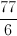Therefore, the short side of the rectangle is going to be: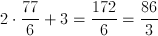And the long side is going to be: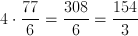The area of the rectangle is going to be as follows:

Area = lw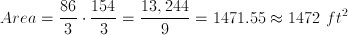### Example Question #9 : How To Find The Area Of A Rectangle

The area of a rectangle is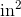and its perimeter is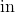. What are its dimensions?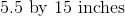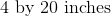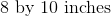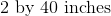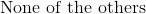Explanation:

Based on the information given to you, you know that the area could be written as: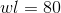Likewise, you know that the perimeter is: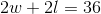Now, isolate one of the values. For example, based on the second equation, you know: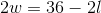Dividing everything by, you get: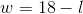Now, substitute this into the first equation: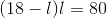To solve for, you need to isolate all of the variables on one side: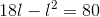or: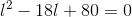Now, factor this: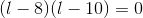, meaning thatcould be eitheror. These are the dimensions of your rectangle.

You could also get this answer by testing each of your options to see which one works for both the perimeter and the area.

### Example Question #10 : How To Find The Area Of A Rectangle

A rectangle having a width twice the length of its height has an area of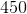. What is the length of its longer side?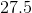Explanation:

Since the width is twice the height, we know that the general area equation, which is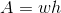could be written: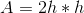Thus, we know: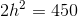or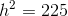This means thatmust be; however, notice that the question asks for the length of the longer side. Thus, the answer is.

← Previous 1